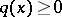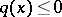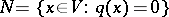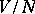# Semi-definite form

(diff) ← Older revision | Latest revision (diff) | Newer revision → (diff)
A quadratic formover an ordered field which represents either only non-negative field elements or only non-positive field elements. In the former case the form is said to be a non-negative definite (for all), in the latter case — a non-positive definite quadratic form (). Most frequently one considers semi-definite forms over the fieldof real numbers. For the fielda similar definition yields the concept of (non-negative and non-positive) semi-definite Hermitian quadratic forms (see Hermitian form).
Ifis a symmetric bilinear (cf. Bilinear form) or Hermitian form such thatis a semi-definite form, thenis sometimes also called a (non-negative or non-positive) semi-definite form. Ifis a quadratic or Hermitian semi-definite form in a vector space, thenis a subspace, identical with the kernel of, and the given form naturally induces a positive-definite or negative-definite form on.